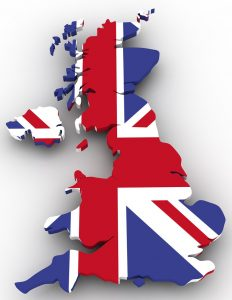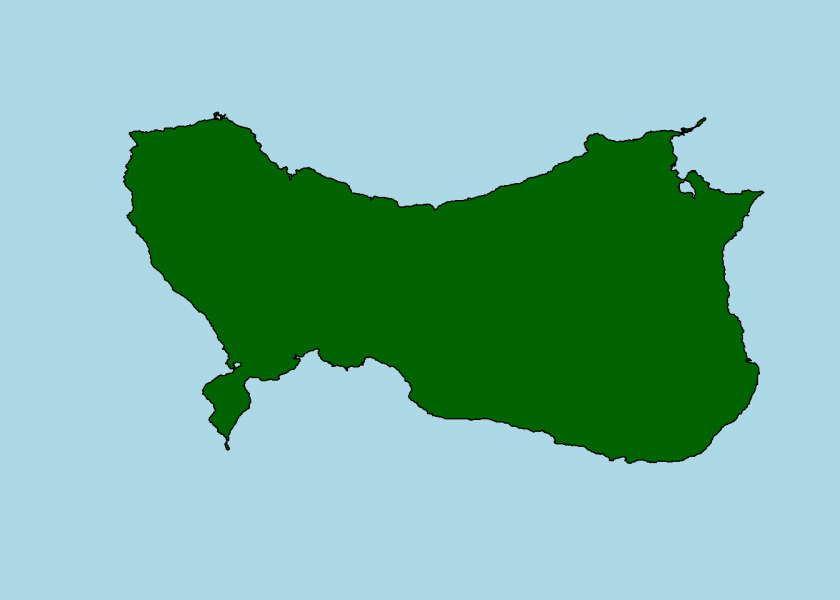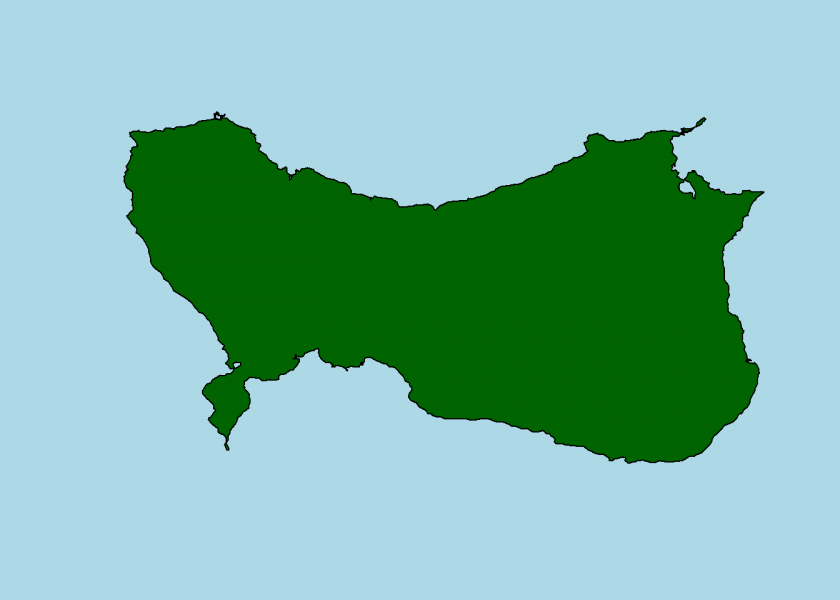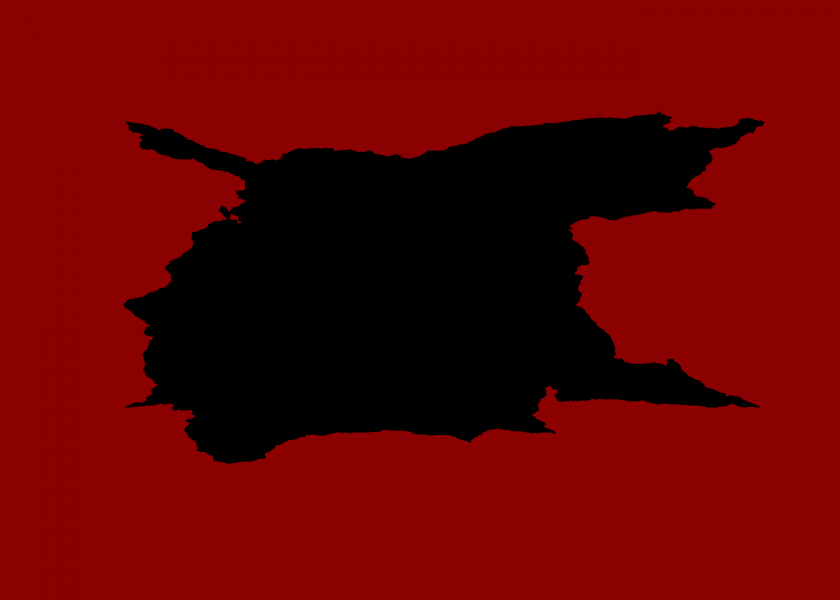Want to share your content on R-bloggers? click here if you have a blog, or here if you don't.Modern movies use a lot of mathematics for their computer animations and CGI effects, one of them is what is known under the name fractals.

In this post, we will use this technique to create islands with coastlines that look extraordinarily realistic. If you want to do that yourself read on!

I was lucky enough to meet Professor Benoit Mandelbrot, one of the brightest minds of the past century and one of the fathers of fractal geometry, in person. One of his papers bears the title “How Long is the Coast of Britain?” where the so-called coastline paradox is being addressed: it basically states that the finer your ruler is the longer your overall result will be, so the coastline of a landmass does not have a well-defined length… an obviously counterintuitive result:

Here we will turn this principle on its head and use it to actually create realistic-looking landmasses with R. The inspiration for this came from chapter 4 “Infinite Detail” of the book “Math Bytes” by my colleague Professor T. Chartier from Davidson College in North Carolina.

The idea is to start with some very simple form, like a square, and add more detail step-by-step. Concretely, we go through every midpoint of our ever more complex polygon and shift it by a random amount. Because the polygon will be getting more and more intricate we have to adjust the absolute amount by which we shift the respective midpoints. That’s about all… have a look at the following code:

# define square
x <- c(0, 16, 16, 0, 0)
y <- c(0, 0, 16, 16, 0)

# function for generating random offsets of midpoints, change e.g. limit for your own experiments
realistic <- function(n, k) {
limit <- 0.5^k * 10
runif(n, -limit, limit)
}

# function for calculating all midpoints of a polygon
midpoints <- function(x) {
na.omit(filter(x, rep(1/2, 2)))
}

island <- function(x, y, iter = 10, random = realistic) {
# suppress "number of columns of result is not a multiple of vector length"-warnings because recycling is wanted here
oldw <- getOption("warn")
options(warn = -1)

for (i in 1:iter) {
# calculate midpoints of each line segment
x_m <- as.numeric(midpoints(x))
y_m <- as.numeric(midpoints(y))

# shift midpoint by random amount
x_m <- x_m + random(length(x_m), i)
y_m <- y_m + random(length(y_m), i)

#insert new midpoints into existing coordinates of polygon
x_n <- c(rbind(x, x_m))
x_n <- x_n[-length(x_n)]
y_n <- c(rbind(y, y_m))
y_n <- y_n[-length(y_n)]

x <- x_n
y <- y_n
}
options(warn = oldw)
list(x = x, y = y)
}

plot_island <- function(coord, island_col = "darkgreen", water_col = "lightblue") {
oldp <- par(bg = water_col)
plot(coord$x, coord$y, type = "n", axes = FALSE, frame.plot = FALSE, ann = FALSE)
polygon(coord$x, coord$y, col = island_col)
par(oldp)
}


Perhaps one thing is worth mentioning concerning the interaction of the functions: island receives the realistic function as an argument, which is a very elegant way of modularizing the code in case you want to try different randomizing functions. This extraordinary feature is possible because R is a functional programming language where functions are first-class citizens (for an introduction on this see here: Learning R: A gentle introduction to higher-order functions).

But now for some serious terra-forming:

set.seed(1) # for reproducability
coord <- island(x, y)
plot_island(coord)set.seed(3)
coord <- island(x, y)
plot_island(coord)We can also observe the process step-by-step:

set.seed(2)
coord <- island(x, y)
plot_island(coord)set.seed(2)
coord <- island(x, y, iter = 1)
plot_island(coord)set.seed(2)
coord <- island(x, y, iter = 2)
plot_island(coord)set.seed(2)
coord <- island(x, y, iter = 4)
plot_island(coord)set.seed(2)
coord <- island(x, y, iter = 6)
plot_island(coord)set.seed(2)
coord <- island(x, y, iter = 8)
plot_island(coord)If you do your own experiments with the randomizer function and/or the seed and find an especially appealing example, please share it with us in the comments!

As a bonus, I would like to draw your attention to this little masterpiece of an educational film on fractals, created in the style of the great “Sin City” flick (~ 5 min.):

So, let me end this post with one last example of a volcanic island in a sea of lava…set.seed(5214)
coord <- island(x, y)
plot_island(coord, island_col = "black", water_col = "darkred")